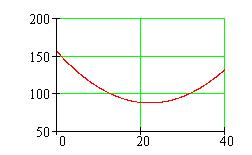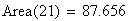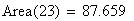Problem
A wire 50 inches long is cut into two pieces. One piece is bent into a circle; the other, into a square. Where should the wire be cut to minimize the sum of the areas of the two shapes?
Solution
Suppose the cut gives x inches to the circle and 50 – x inches for the square. Then we can compute the areas of the shapes in terms of the unknown length x.
Circle
Square
circumference: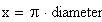perimeter: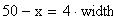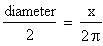width: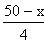area: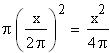area: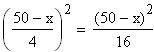The total of the areas is a function of x.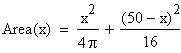Since we want to minimize this function, we take its derivative, set it equal to zero, and solve for x.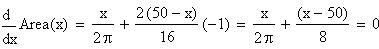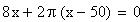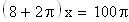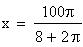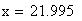This answer is accurate to 3 decimal places.
Therefore, to minimize the sum of the areas of the shapes, the wire should be cut into pieces approximately 21.995 inches and 28.005 inches long. The combined area will be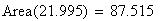We can satisfy ourselves that is is really a minimum by graphing the Area function, and by substituting values slightly smaller and slightly larger than x to see that they give larger total areas.